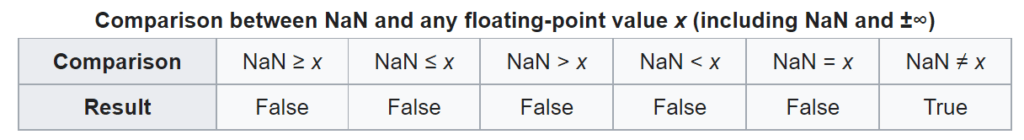# When a double is neither greater nor lesser, is it equal ?When a double is neither greater nor lesser, is it equal ?

This is assumed in some implementations like for RTL’s VarCompareValue and other function that return the result of the comparison.

In those function you can see code like

```else if A = B then
Result := vrEqual
else if A < B then
Result := vrLessThan
else
Result := vrGreaterThan;```

However that code is incorrect when dealing with IEEE double precision floats, for special “Not a Number” values.

The specificity of NaN is that is is different from everything else:So when dealing with floats, you have to go the whole way

```else if A = B then
Result := vrEqual
else if A < B then
Result := vrLessThan
else if A > B then
Result := vrGreaterThan
else
Result := vrNotEqual;```

## 3 thoughts on “When a double is neither greater nor lesser, is it equal ?”

1. But comparing NaN with a number should raise an exception anyway, should it not?

2. Thorsten Engler says:

The Delphi RTL is written under the assumption that floating point exceptions are not masked. In which case , yes, it will raise an exception.

If you mask floating point exceptions, Delphi is going to misbehave in various ways. I’m not sure in how far this is documented, but basically, you shouldn’t normally run delphi code with masked floating point exceptions.

3. @Thorsten actually that’s the first issue related to floating point exception masking I’ve encountered since using Delphi (back with Delphi 3), and it only occurred because a variant ended up being used by accident.

The reason not use FPU masking is both for coherence with other environments (where it’s the norm) and easier debuggability when doing computations on datasets. It’s way easier to track the root cause of a few NaN in a huge dataset than provide enough context in the exception frame to figure out why a floating point exception occurred, as that context can be a whole lot of input data.